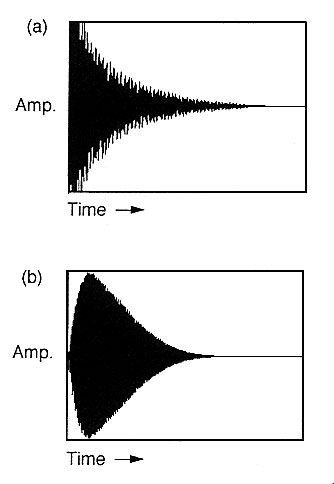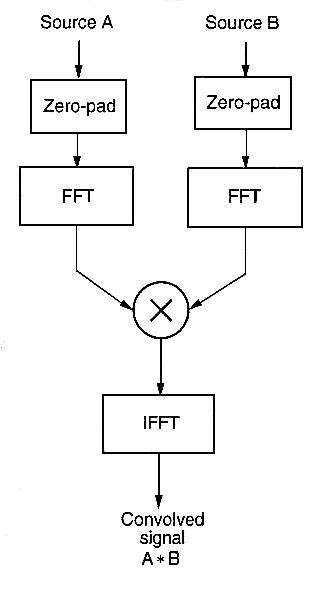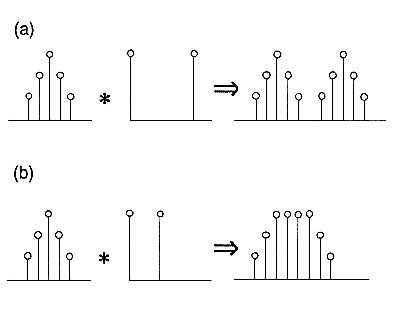# Convolution

Convolution has been a standard topic in engineering and computing science for some time, but only since the early 1990s has it been widely available to computer music composers, thanks largely to the theoretical descriptions by Curtis Roads (1996), and the SoundHack software of Tom Erbe that made this technique accessible.

Convolving two waveforms in the time domain means that you are multiplying their spectra (i.e. frequency content) in the frequency domain. By "multiplying" the spectra we mean that any frequency that is strong in both signals will be very strong in the convolved signal, and conversely any frequency that is weak in either input signal will be weak in the output signal.

 TIME DOMAIN FREQUENCY DOMAIN CONVOLUTION < ------------------------------ > MULTIPLICATION MULTIPLICATION < ------------------------------ > CONVOLUTION

In practice, a relatively simple application of convolution is where we have the "impulse response" of a space. This is obtained by recording a short burst of a broad-band signal as it is processed by the reverberant characteristics of the space. When we convolve any "dry" signal with that impulse response, the result is that the sound appears to have been recorded in that space. In other words, it has been processed by the frequency response of the space similar to how that process would work in the actual space. In fact, convolution in this example is simply a mathematical description of what happens when any sound is "coloured" by the acoustic space within which it occurs, which is in fact true of all sounds in all spaces except an anechoic chamber. The convolved sound will also appear to be at the same distance as in the original recording of the impulse. If we convolve a sound twice with the same impulse response, its apparent distance will be twice as far away.

For instance, in a reverberant space, one might clap one's hands to get a sense of the acoustics of the space. However, a more accurate impulse response would be obtained by firing a starter pistol, as that sound's spectrum would be more evenly distributed and the sound is very short. Given the intrusiveness of such an action as firing a gun, a more acceptable approach would be breaking a balloon and recording the response of the space.

In fact, one can convolve any sound with another, not just an impulse response. In that case, we are "filtering" the first sound through the spectrum of the second, such that any frequencies the two sounds have in common will be emphasized. A particular case is where we convolve the sound with itself, thereby guaranteeing maximum correlation between the two sources. In this case, prominent frequencies will be exaggerated and frequencies with little energy will be attenuated.

However, the output duration of a convolved signal is the sum of the durations of the two inputs. With reverberation we expect the reverberated signal to be longer than the original, but this extension and the resultant "smearing" of attack transients also occurs when we convolve a sound with itself, or with another sound. Transients are smoothed out, and the overall sound is lengthened (by a factor of two in the case of convolving a sound with itself). When we convolve this stretched version with the impulse response of a space, the result appears to be half way between the original and the reverberant version, a "ghostly" version of the sound, so to speak.Smearing of attack transients

Since most acoustic sounds (but not common electronic and digital sounds, unfortunately) have spectra that taper off with increasing frequency, the high frequencies may be weak when convolved with a spectrum with similar characteristics. Therefore, some programs such as SoundHack allow the high frequencies to be boosted during convolution. This can also result in the result being "hissy" and therefore equalization needs to be applied.

The inverse of convolving two waveforms is multiplying them, as in ring modulation. In this case we are convolving their spectra which is why ring modulation results in the sum and difference frequencies of each component being present in the output, though an understanding of this result depends on the mathematics of the complex domain. In other words, the basic theorem about the time domain and the frequency domain is that multiplication in one domain is equivalent to convolution in the other domain.

Finally, there is a technical difference between "direct convolution", which is a very slow process given that every sample in each signal must be multipled by every sample in the other signal, and the faster version used by programs like SoundHack which analyzes each signal using an FFT (Fast Fourier Transform) then multiplying those results and performing the Inverse FFT to return the result to the time domain. Besides increasing the speed of the calculation (thereby bringing it into a reasonable working process), other variables involved in the analysis phase are brought into play, such as the window shape used in the analysis. However, in practice, this variable only affects the result quite subtly. If a rectangular window is used, convolving A * B is exactly the same as B * A (note that the * symbol is used to denote convolution, as opposed to multiplication). However, other curved forms of windows result in a difference between the two versions, mainly the overall amplitude shape of the result.Convolving a signal with two impulses farther apart and closer together

Convolution has been used extensively in Barry Truax's work Temple, The Shaman Ascending, The Way of the Spirit, Chalice Well, From the Unseen World, Aeolian Voices, Earth & Steel, The Garden of Sonic Delights, The Bells of Salzburg, and Infinity Room. Sound examples are provided on the author's DVD-ROM and the WSP Database. Additional discussion in this paper and practical strategies including auto-convolution (i.e. convolving a sound with itself) and convolving independent sounds are discussed in this tutorial article.

Reference:

C. Roads, The Computer Music Tutorial, MIT Press, 1996

home